# Shortest Path Algorithm Dijkstras Algorithm BellmanFord Algorithm 8

• Slides: 19Shortest Path Algorithm • Dijkstra’s Algorithm • Bellman-Ford Algorithm 8 5 3 2 6 2 3 2 1 3 2 4 6 8 3 3 1 1 7 5 1 1 1 4 2 5 Packet Switching 1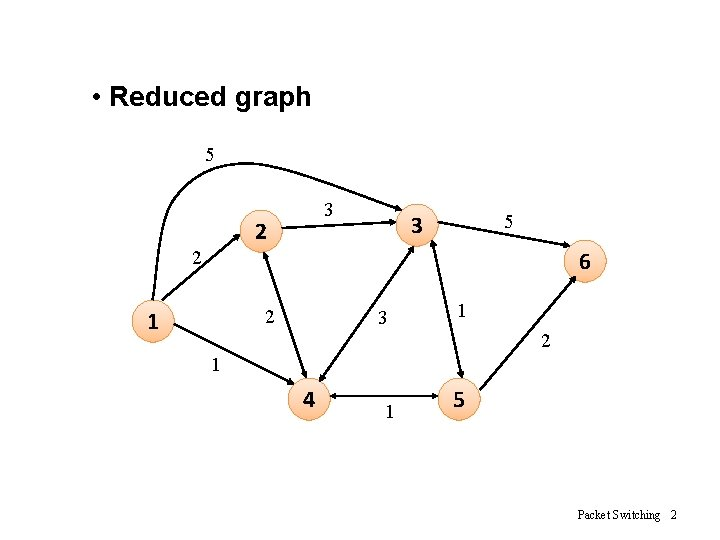• Reduced graph 5 3 2 3 5 6 2 2 1 3 1 2 1 4 1 5 Packet Switching 2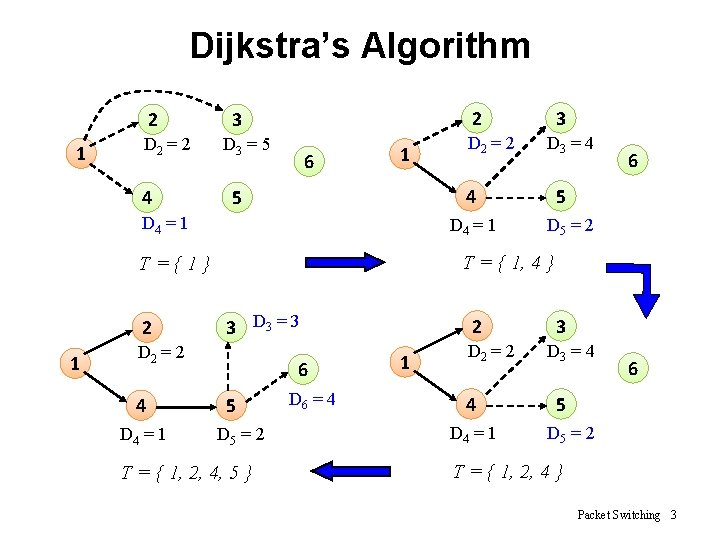Dijkstra’s Algorithm 2 1 D 2 = 2 4 2 3 D 3 = 5 6 1 D 4 = 1 3 D 3 = 3 D 2 = 2 4 D 4 = 1 6 5 D 5 = 2 T = { 1, 4 } T={1} 1 D 3 = 4 4 5 D 4 = 1 2 D 2 = 2 3 6 5 D 5 = 2 T = { 1, 2, 4, 5 } D 6 = 4 2 1 D 2 = 2 4 D 4 = 1 3 D 3 = 4 6 5 D 5 = 2 T = { 1, 2, 4 } Packet Switching 3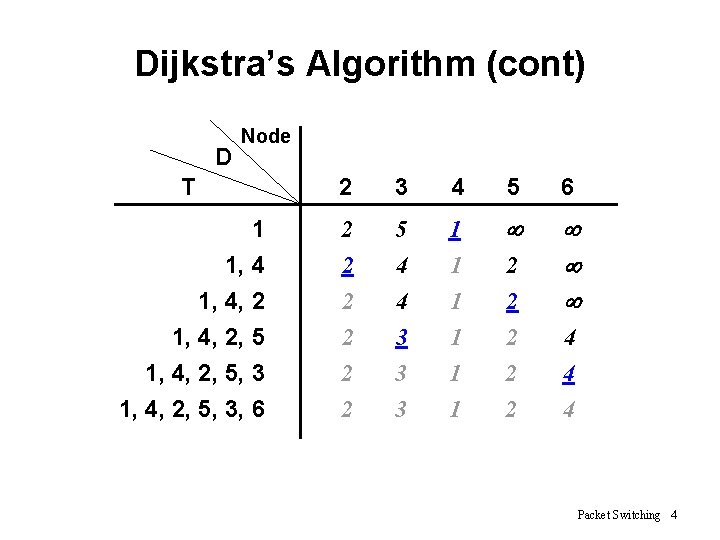Dijkstra’s Algorithm (cont) D Node T 2 3 4 5 6 1 1, 4, 2, 5, 3 1, 4, 2, 5, 3, 6 2 5 1 ¥ 2 2 2 4 4 3 3 3 1 1 1 2 2 2 ¥ ¥ ¥ 4 4 4 Packet Switching 4Dijkstra’s Algorithm (cont) • w(i, j) = link cost, L(n) = path cost from node s to n • 1. [Initialization] – T = {s} – L(n) = w(s, n) for n ≠ s • 2. [Get next node] – Find x Ï T such that L(x) = min L(j) jÏT – Add x to T • 3. [Update Least-Cost Paths] – L(n) = min [ L(n), L(x) + w(x, n) ] for all n Ï T – Go to step 2 Packet Switching 5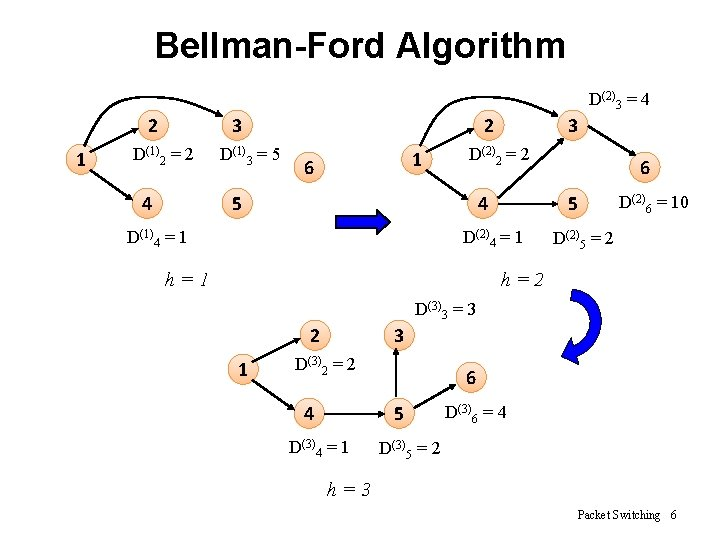Bellman-Ford Algorithm D(2)3 = 4 2 1 3 D(1)2 = 2 4 D(1)3 = 5 2 1 6 3 D(2)2 = 2 5 4 D(1)4 = 1 5 D(2)4 = 1 h=1 6 D(2)6 = 10 D(2)5 = 2 h=2 D(3)3 = 3 2 1 3 D(3)2 = 2 4 6 5 D(3)4 = 1 D(3)6 = 4 D(3)5 = 2 h=3 Packet Switching 6Bellman-Ford Algorithm (cont) D Node h Source = 1 0 1 2 3 4 5 6 ¥ ¥ ¥ 2 5 1 ¥ ¥ 2 2 2 4 3 3 1 1 1 2 2 2 10 4 4 Packet Switching 7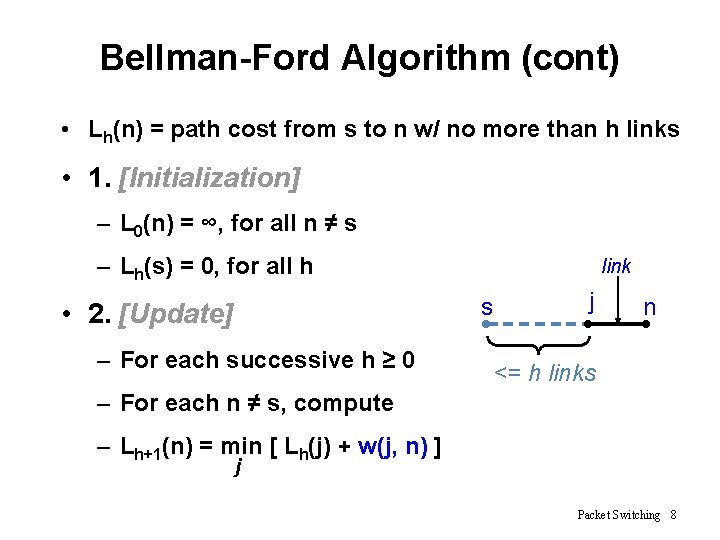Bellman-Ford Algorithm (cont) • Lh(n) = path cost from s to n w/ no more than h links • 1. [Initialization] – L 0(n) = ∞, for all n ≠ s – Lh(s) = 0, for all h link j s • 2. [Update] – For each successive h ≥ 0 n <= h links – For each n ≠ s, compute – Lh+1(n) = min [ Lh(j) + w(j, n) ] j Packet Switching 8Comparisons L(n) = min [ L(n), L(x) + w (x, n) ] Lh(x, D) x S … D S x …… D x Dijkstra’s (Link State) Bellman-Ford (Distance Vector) Packet Switching 9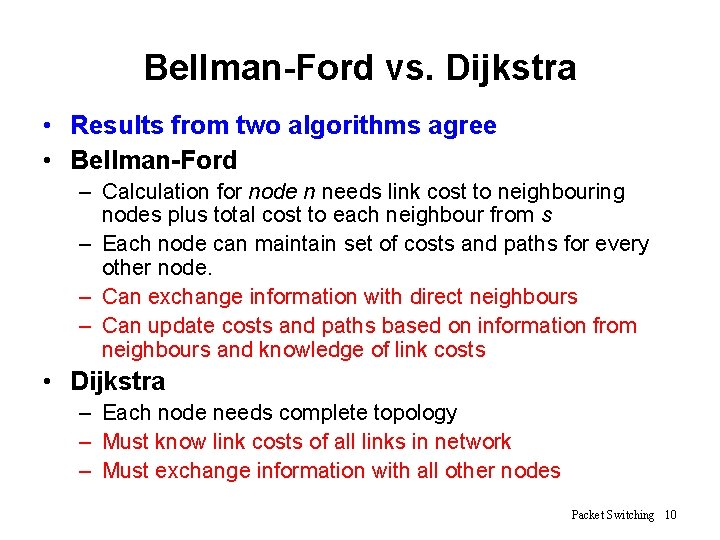Bellman-Ford vs. Dijkstra • Results from two algorithms agree • Bellman-Ford – Calculation for node n needs link cost to neighbouring nodes plus total cost to each neighbour from s – Each node can maintain set of costs and paths for every other node. – Can exchange information with direct neighbours – Can update costs and paths based on information from neighbours and knowledge of link costs • Dijkstra – Each node needs complete topology – Must know link costs of all links in network – Must exchange information with all other nodes Packet Switching 10Routing in ARPANET • First generation(RIP), 1969 – Adaptive Routing is adopted – Use Bellman-Ford algorithm Distance Vector – Estimated link delay is simply the queue length for that link – Every 128 ms, each node exchanges its delay vector (routing table) with all its neighbors – Information about a change in network condition would gradually ripple through the network Packet Switching 11Routing in ARPANET (cont) – Each node i maintains • di j = current estimate of min delay from i to j • si j = next node in the current min-delay route from i to j – Node k updates its vectors as follows • dk j = Min [ lk i + di j ] iÎA • sk j = i k i j using i that minimizes the expression above where A = set of neighbor nodes for k lk i = current estimate of delay from k to i Packet Switching 12Routing in ARPANET (cont) • Major shortcomings of RIP – It did not consider line speed, merely queue length. Higher capacity links were not given the favored status – Queue length is an artificial measure of delay – The algorithm was not very accurate. It responded slowly to congestion and delay increases. Packet Switching 13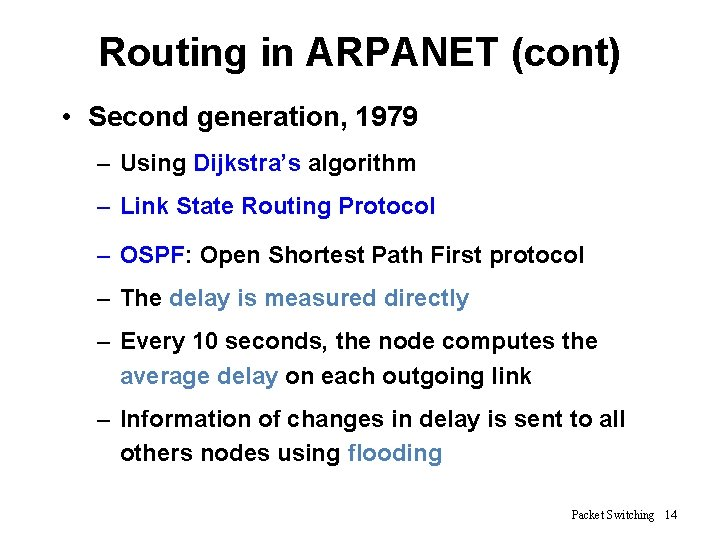Routing in ARPANET (cont) • Second generation, 1979 – Using Dijkstra’s algorithm – Link State Routing Protocol – OSPF: Open Shortest Path First protocol – The delay is measured directly – Every 10 seconds, the node computes the average delay on each outgoing link – Information of changes in delay is sent to all others nodes using flooding Packet Switching 14Routing in ARPANET (cont) Packet Switching 15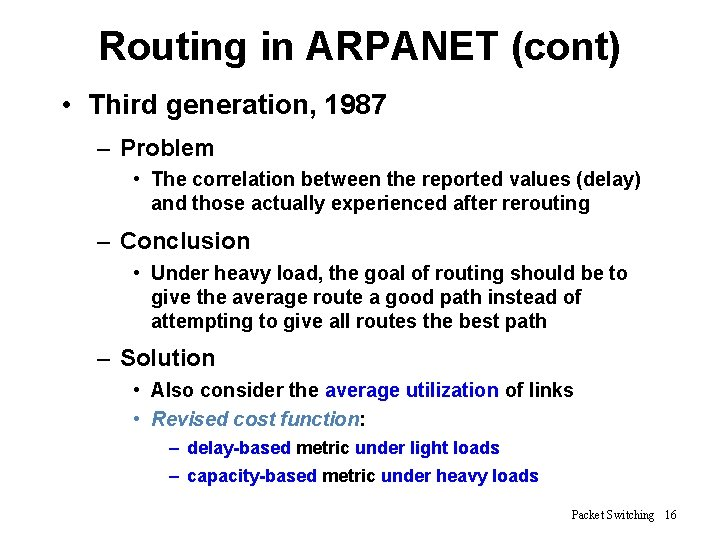Routing in ARPANET (cont) • Third generation, 1987 – Problem • The correlation between the reported values (delay) and those actually experienced after rerouting – Conclusion • Under heavy load, the goal of routing should be to give the average route a good path instead of attempting to give all routes the best path – Solution • Also consider the average utilization of links • Revised cost function: – delay-based metric under light loads – capacity-based metric under heavy loads Packet Switching 16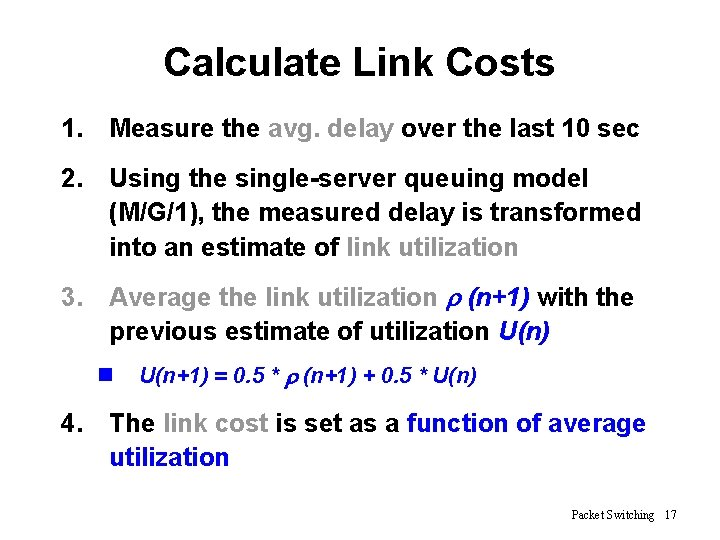Calculate Link Costs 1. Measure the avg. delay over the last 10 sec 2. Using the single-server queuing model (M/G/1), the measured delay is transformed into an estimate of link utilization 3. Average the link utilization r (n+1) with the previous estimate of utilization U(n) n U(n+1) = 0. 5 * r (n+1) + 0. 5 * U(n) 4. The link cost is set as a function of average utilization Packet Switching 17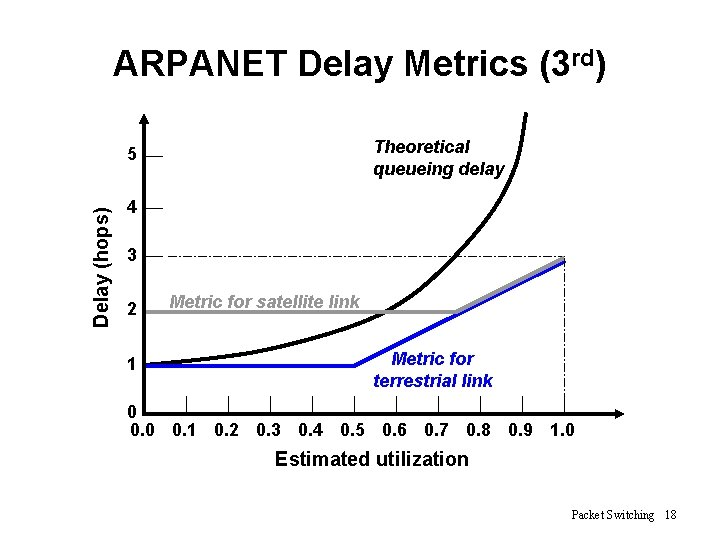ARPANET Delay Metrics (3 rd) Theoretical queueing delay Delay (hops) 5 4 3 2 1 Metric for satellite link Metric for terrestrial link 0 0. 1 0. 2 0. 3 0. 4 0. 5 0. 6 0. 7 0. 8 0. 9 1. 0 Estimated utilization Packet Switching 18ARPANET Delay Metrics (3 rd) 1. Delay is normalized to the value achieved on an idle line 2. The cost value is kept at the minimum value until a given level of utilization is reached Ø Reducing routing overhead at low traffic levels 3. Above a certain level of utilization, the cost level is allowed to rise to a maximum value that is equal to three times the minimum value Ø To dictate that traffic should not be routed around a heavily utilized line by more than two additional hops Packet Switching 19